### Magnetism And Matter Class 12th Physics Part I CBSE Solution

##### Question 1.Answer the following question:Why does a paramagnetic sample display greater magnetization (for the same magnetizing field) when cooled?Answer:At high temperatures, due to the random thermal motion of molecules in a paramagnetic sample, the alignments of dipoles get disrupted. On reducing the temperature random thermal motion reduces, thus disruption is reduced. Hence, a paramagnetic sample displays greater magnetization when cooled.Question 2.Answer the following question:Why is diamagnetism, in contrast, almost independent of temperature?Answer:The magnetism in a diamagnetic substance is due to induced dipole moment (opposite to the magnetizing field). Hence, the internal motion of the atoms, which depends on the temperature, does not affect the diamagnetism of a material.Question 3.Answer the following question:If a toroid uses bismuth for its core, will the field in the core be (slightly) greater or (slightly) less than when the core is empty?Answer:A toroid using bismuth core has a magnetic field slightly greater than a toroid whose core is empty because bismuth is a diamagnetic substance.Question 4.Answer the following question:Is the permeability of a ferromagnetic material independent of the magnetic field? If not, is it more for lower or higher fields?Answer:The permeability of ferromagnetic materials is inversely dependent on the applied magnetic field, i.e. it is lower for a higher field and vice versa.Question 5.Answer the following question:Magnetic field lines are always nearly normal to the surface of a ferromagnet at every point. (This fact is analogous to the static electric field lines being normal to the surface of a conductor at every point.) Why?Answer:The magnetic field lines are always nearly normal to the surface of ferromagnetic materials at every point because permeability of ferromagnetic material is always greater than one.Question 6.Answer the following question:Would the maximum possible magnetization of a paramagnetic sample be of the same order of magnitude as the magnetization of a ferromagnet?Answer:Yes, the maximum possible magnetization of a paramagnetic sample can be of the same order of magnitude as the magnetization of a ferromagnet but this requires very strong magnetic fields.Question 7.Answer the following question:Explain qualitatively on the basis of domain picture the irreversibility in the magnetization curve of a ferromagnet.Answer:The relation between B (i.e. the external magnetic field) and H (i.e. magnetic intensity) in ferromagnetic materials is shown in the following curve.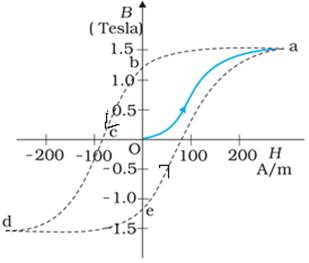From the above curve it is clear that magnetization persists even when the external field is removed, this depicts the irreversibility of magnetization in a ferromagnet.Question 8.Answer the following question:The hysteresis loop of a soft iron piece has a much smaller area than that of a carbon steel piece. If the material is to go through repeated cycles of magnetization, which piece will dissipate greater heat energy?Answer:After going through repeated cycles of magnetization, a carbon steel piece dissipates greater heat energy than a soft iron piece, because the dissipated heat energy is directly proportional to the area of a hysteresis loop and the carbon steel piece has a greater hysteresis curve area than a soft iron piece.Question 9.Answer the following question:‘A system displaying a hysteresis loop such as a ferromagnet, is a device for storing memory?’ Explain the meaning of this statement.Answer:The record of hysteresis loop cycles during magnetization (i.e. value of magnetization) corresponds to bits of information. Therefore, the material displaying hysteresis loops like a ferromagnet can be used for storing information.Question 10.Answer the following question:What kind of ferromagnetic material is used for coating magnetic tapes in a cassette player, or for building ‘memory stores’ in a modern computer?Answer:Ferromagnetic material like Ceramic is used for coating magnetic tapes in cassette players and for building memory stores in modern computers.Question 11.Answer the following question:A certain region of space is to be shielded from magnetic fields. Suggest a method.Answer:The certain region of space can be surrounded by soft iron coil to shield it from magnetic field.Question 12.A long straight horizontal cable carries a current of 2.5 A in the direction 10° south of west to 10° north of east. The magnetic meridian of the place happens to be 10° west of the geographic meridian. The earth’s magnetic field at the location is 0.33 G, and the angle of dip is zero. Locate the line of neutral points (ignore the thickness of the cable)? (At neutral points, magnetic field due to a current-carrying cable is equal and opposite to the horizontal component of earth’s magnetic field.)Answer:Current in the cable (I) = 2.5 AEarth’s magnetic field at the location (H) = 0.33 G = 0.33 × 10-4TAngle of dip (δ) = 0Let the line of neutral points be at a distance “r” meters from the horizontal cable.The magnetic field at the neutral point due to current carrying cable,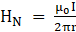,where μ0 = Permeability of free space = 4π × 10-7 TmA1Horizontal component of earth’s magnetic field (H’) = H × cosδAt neutral points, magnetic field due to a current-carrying cable is equal and opposite to the horizontal component of earth’s magnetic field.∴ H’ = HN⇒ H × cosδ =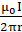⇒ 0.33 × 10-4 T × cos0o =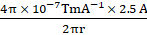⇒ r =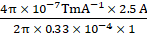⇒ r = 15.15 × 10-3m⇒ r = 1.515cmQuestion 13.A telephone cable at a place has four long straight horizontal wires carrying a current of 1.0 A in the same direction east to west. The earth’s magnetic field at the place is 0.39 G, and the angle of dip is 35°. The magnetic declination is nearly zero. What are the resultant magnetic fields at points 4.0 cm below the cable?Answer:Current in the wires (I) = 1.0 A (east to west)The earth’s magnetic field at the place (H) = 0.39G = 0.39 × 10-4TThe angle of dip (δ) = 35°Magnetic declination (θ) ∼ 0oNumber of wires in the cable (n) = 4The horizontal component of earth’s magnetic field (H’) = H × cosδMagnetic fields due to current carrying cables (B) =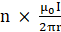where μ0 = Permeability of free space = 4π × 10-7TmA1The resultant magnetic fields at point 4.0 cm below the cablesR = 4cm = 0.04mResultant horizontal magnetic fields (Hh) = H’- B⇒ Hh = H’- B⇒ Hh =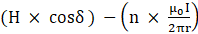⇒ H � �h = (0.39 × 10-4T × cos35o)-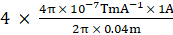⇒ Hh = 0.39 × 10-4T × 0.819 - 4 × 2 × 25 × 10-7T⇒ Hh = 0.319 × 10-4T-0.2 × 10-4T⇒ Hh = 0.119 × 10-4Or Hh ∼ 0.12 × 10-4 T or 0.12 GaussResultant vertical magnetic field (H � �v �) = vertical component of earth’s magnetic field = H × sinδ= 0.39 × 10-4T × sin35o= 0.39 × 10-4T × 0.573= 0.22 × 10-4T∴ Resultant magnetic field =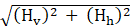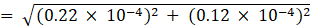= 0.25 × 10-4TQuestion 14.A compass needle free to turn in a horizontal plane is placed at the centre of circular coil of 30 turns and radius 12 cm. The coil is in a vertical plane making an angle of 45° with the magnetic meridian. When the current in the coil is 0.35 A, the needle points west to east.(a) Determine the horizontal component of the earth’s magnetic field at the location.(b) The current in the coil is reversed, and the coil is rotated about its vertical axis by an angle of 90° in the anticlockwise sense looking from above. Predict the direction of the needle. Take the magnetic declination at the places to be zero.Answer:(a) Number of turns in the coil (n) = 30Radius of coil (r) = 12cm = 0.12mCurrent in the coil (I) = 0.35AAngle of dip (δ) = 45oMagnetic fields due to current carrying coils (B) =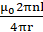where, μ0 = Permeability of free space = 4π × 10-7TmA1B =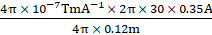= 5.49 × 10-5 T∴ Horizontal component of the earth’s magnetic field = B sinδ= 5.49 × 10-5 T × sin45o= 5.49 × 10-5 T × 0.707= 3.88 × 10-5 T(b) When the current in the coil is reversed, and the coil is rotated about its vertical axis by an angle of 90° in the anticlockwise sense looking from above, then the needle will reverse its original direction (i.e. the needle will point from East to West).Question 15.A magnetic dipole is under the influence of two magnetic fields. The angle between the field directions is 60°, and one of the fields has a magnitude of 1.2 × 10–2 T. If the dipole comes to stable equilibrium at an angle of 15° with this field, what is the magnitude of the other field?Answer:Magnitude of one of the magnetic fields (B1) = 1.2 × 10–2 TThe angle between the magnetic field directions (θ) = 60°The angle between the dipole and the magnetic field B1 is θ1 = 15°Let magnitude of second of the magnetic fields = B2∴ The angle between the dipole and the magnetic field B2 is θ2 = θ-θ1 = 45°Let magnetic moment of the dipole be MThus, at rotational equilibrium,Torque due to field B1 = Torque due to field B2⇒ M × B1sinθ1 = M × B2 sinθ2⇒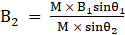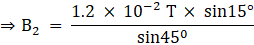⇒ B2 = 9 × 10-3 TThus, the magnitude of the other magnetic field = 4.39 × 10-3TQuestion 16.A monoenergetic (18 keV) electron beam initially in the horizontal direction is subjected to a horizontal magnetic field of 0.04 G normal to the initial direction. Estimate the up or down deflection of the beam over a distance of 30 cm (me = 9.11 × 10–31 kg). [Note: Data in this exercise are so chosen that the answer will give you an idea of the effect of earth’s magnetic field on the motion of the electron beam from the electron gun to the screen in a TV set.]Answer:Energy of the electron beam(E) = 18keV = 18000eV = 18000 × 1.6 × 10-19 = 2.88 × 10-15JMass of electron (me) = 9.11 × 10–31 kgHorizontal magnetic field (B) = 0.04 G = 0.04 × 10-4TDistance of deflection from the beam (r) = 30 cm = 0.3mLet velocity of electrons be v.∴ Energy of the beam = Kinetic energy of electrons⇒ E = 1/2 mev2⇒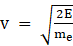⇒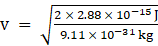∴ v = 7.95 × 107m/s∵ The electron beam deflects along a circular path in a magnetic field.Let the radius of the circular path be r m.The force due to magnetic field on the electron = qvBsinθ = e × v × B × sin 90°Here, q = charge on the electron,θ = angle between velocity and magnetic field.∵ The force due to magnetic field on electron = centripetal force on electron⇒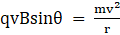⇒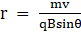⇒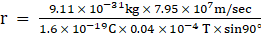⇒ r = 11.3 mLet the deflection of electron beam in upward and downward directions be x = r(1-cosΦ),where Φ = angle of deflection.∵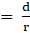⇒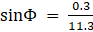= 0.026⇒ Φ = sin-1(0.026) = 1.521°Thus, Deflection(x) = r(1- cosΦ) = 11.3 × (1- cos(1.521)°)= 11.3 × (1- 0.90)= 11.3 × 0.01= 1.1310-3mQuestion 17.A sample of paramagnetic salt contains 2.0 × 1024 atomic dipoles each of dipole moment 1.5 × 10–23 J T–1. The sample is placed under a homogeneous magnetic field of 0.64 T, and cooled to a temperature of 4.2 K. The degree of magnetic saturation achieved is equal to 15%. What is the total dipole moment of the sample for a magnetic field of 0.98 T and a temperature of 2.8 K? (Assume Curie’s law)Answer:Number of atomic dipoles (n) = 2 × 1024Initially, Dipole moment of each atomic dipole (M) = 1.5 × 10-23JT-1Magnetic field (B1) = 0.64 T and Temperature (θ1) = 4.2K∵ Degree of magnetic saturation = 15%∴ Effective Dipole moment (M1) = 15% of Total dipole moment⇒ M1 =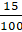× (no. of atomic dipole × dipole moment of each)⇒ M1 =× 2 × 1024 × 1.5 × 10-23JT-1 = 4.5 JT-1When, Magnetic field (B2) = 0.98 T and Temperature (θ2) = 2.8 KLet the dipole moment be M2.According to the Curie’s Law, magnetization of a paramagnetic material is directly proportional to applied magnetic field and inversely proportional to temperature.∴ Ratio of magnetic dipole moments (i.e. M1:M2) = (B1θ2/B2θ1)⇒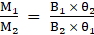⇒ M2 = M1 ×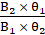⇒ M2 = 4.5 JT-1 ×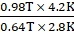= 10.336 JT-1Thus, total dipole moment of the sample for a magnetic field of 0.98 T and a temperature of 2.8 K = 10.336 JT-1Question 18.A Rowland ring of mean radius 15 cm has 3500 turns of wire wound on a ferromagnetic core of relative permeability 800. What is the magnetic field B in the core for a magnetising current of 1.2 A?Answer:Radius of ring (r) = 15cm = 0.15mNumber of turns in the ring (n) = 3500Relative permeability of the ferromagnetic core (μr) = 800Current in the Rowland ring (I) = 1.2AMagnetic Field due to circular coil (B) =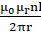Where, μ0 = Permeability of free space = 4π × 10-7TmA-1∴ B =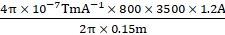= 4.48TQuestion 19.The magnetic moment vectors μs and μl associated with the intrinsic spin angular momentum S and orbital angular momentum l, respectively, of an electron are predicted by quantum theory (and verified experimentally to a high accuracy) to be given by:μs = – (e/m) S,μl = – (e/2m)lWhich of these relations is in accordance with the result expected classically? Outline the derivation of the classical result.Answer:We know,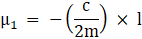It follows from the definition of μ1 and l.μ1 = i × A =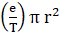l = mvr = m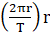Where, r is the radius of the circular path traced by the electron around the nucleus, with the mass of electron m and charge (-e) and time period T.Divide the above equations-⇒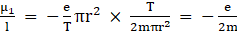⇒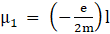Clearly μ1 and l will be antiparallel.On the other hand,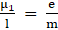is obtained on the basis of quantum mechanics.

PDF FILE TO YOUR EMAIL IMMEDIATELY PURCHASE NOTES & PAPER SOLUTION. @ Rs. 50/- each (GST extra)

HINDI ENTIRE PAPER SOLUTION

MARATHI PAPER SOLUTION

SSC MATHS I PAPER SOLUTION

SSC MATHS II PAPER SOLUTION

SSC SCIENCE I PAPER SOLUTION

SSC SCIENCE II PAPER SOLUTION

SSC ENGLISH PAPER SOLUTION

SSC & HSC ENGLISH WRITING SKILL

HSC ACCOUNTS NOTES

HSC OCM NOTES

HSC ECONOMICS NOTES

HSC SECRETARIAL PRACTICE NOTES

# 2019 Board Paper Solution

HSC ENGLISH SET A 2019 21st February, 2019

HSC ENGLISH SET B 2019 21st February, 2019

HSC ENGLISH SET C 2019 21st February, 2019

HSC ENGLISH SET D 2019 21st February, 2019

SECRETARIAL PRACTICE (S.P) 2019 25th February, 2019

HSC XII PHYSICS 2019 25th February, 2019

CHEMISTRY XII HSC SOLUTION 27th, February, 2019

OCM PAPER SOLUTION 2019 27th, February, 2019

HSC MATHS PAPER SOLUTION COMMERCE, 2nd March, 2019

HSC MATHS PAPER SOLUTION SCIENCE 2nd, March, 2019

SSC ENGLISH STD 10 5TH MARCH, 2019.

HSC XII ACCOUNTS 2019 6th March, 2019

HSC XII BIOLOGY 2019 6TH March, 2019

HSC XII ECONOMICS 9Th March 2019

SSC Maths I March 2019 Solution 10th Standard11th, March, 2019

SSC MATHS II MARCH 2019 SOLUTION 10TH STD.13th March, 2019

SSC SCIENCE I MARCH 2019 SOLUTION 10TH STD. 15th March, 2019.

SSC SCIENCE II MARCH 2019 SOLUTION 10TH STD. 18th March, 2019.

SSC SOCIAL SCIENCE I MARCH 2019 SOLUTION20th March, 2019

SSC SOCIAL SCIENCE II MARCH 2019 SOLUTION, 22nd March, 2019

XII CBSE - BOARD - MARCH - 2019 ENGLISH - QP + SOLUTIONS, 2nd March, 2019

# HSCMaharashtraBoardPapers2020

(Std 12th English Medium)

HSC ECONOMICS MARCH 2020

HSC OCM MARCH 2020

HSC ACCOUNTS MARCH 2020

HSC S.P. MARCH 2020

HSC ENGLISH MARCH 2020

HSC HINDI MARCH 2020

HSC MARATHI MARCH 2020

HSC MATHS MARCH 2020

# SSCMaharashtraBoardPapers2020

(Std 10th English Medium)

English MARCH 2020

HindI MARCH 2020

Hindi (Composite) MARCH 2020

Marathi MARCH 2020

Mathematics (Paper 1) MARCH 2020

Mathematics (Paper 2) MARCH 2020

Sanskrit MARCH 2020

Sanskrit (Composite) MARCH 2020

Science (Paper 1) MARCH 2020

Science (Paper 2)

Geography Model Set 1 2020-2021

MUST REMEMBER THINGS on the day of Exam

Are you prepared? for English Grammar in Board Exam.

Paper Presentation In Board Exam

How to Score Good Marks in SSC Board Exams

Tips To Score More Than 90% Marks In 12th Board Exam

How to write English exams?

How to prepare for board exam when less time is left

How to memorise what you learn for board exam

No. 1 Simple Hack, you can try out, in preparing for Board Exam

How to Study for CBSE Class 10 Board Exams Subject Wise Tips?

JEE Main 2020 Registration Process – Exam Pattern & Important Dates

NEET UG 2020 Registration Process Exam Pattern & Important Dates

How can One Prepare for two Competitive Exams at the same time?

8 Proven Tips to Handle Anxiety before Exams!